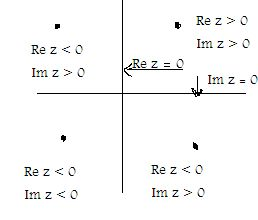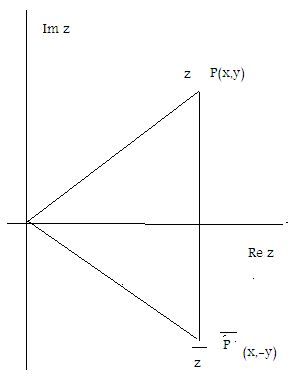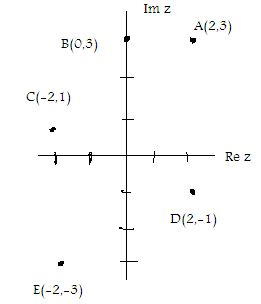Email us to get an instant 20% discount on highly effective K-12 Math & English kwizNET Programs!

#### Online Quiz (WorksheetABCD)

Questions Per Quiz = 2 4 6 8 10

### High School Mathematics - 22.2 Graphical Representation of a Complex Number

Every complex number x+iy can be represented geometrically as a unique point P(x,y) in the XOY plane as shown below with x co-ordiante representing its real part and y-co-ordinate representing the imaginary part.• x- axis is called the real axis and y-axis is called the imaginary axis
• Imaginary numbers with Imz > 0 are represented on the positive y-axis and imaginary numbers with Imz < 0 are represented on the negative y-axis.
This representation of complex numbers as points in the plane is known as the Argand Diagram.
The distance from the origin to the point P(x,y) is defined as the modulus of the complex number z = x+iy and is denoted by |z| shown below
|z| = Öx2+y2
The conjugate z bar of the complex number is represented by the point P bar which is symmetric to P with respect to x-axis.We observe that x = Re z =( z + zbar)/2 y = Im z = (z - z bar)/2 zzbar = |z|2

Example: Represent the complex number 2+i3 by a point in the complex plane.
Solution: The complex number 2+i3 is represented by a point with x-co-ordinate = Re(2+i3) = 2 and y-co-ordinate = Im(2+i3) = 3
The point A(2,3) is located by 2 units on the positive x-axis of real numbers and 3 units on the positive y-axis of imaginary numbers.point B represents imaginary number i3 point C represents -2+i point D represents 2-i point E represents -2-i3

Directions: Plot the following complex numbers in the complex plane.
4-i3
-3+i5
5
2i
-1/2-i3
Ö3/2 +i/2
-4/3 i
1
i
Ö-3

#### Subscription to kwizNET Learning System offers the following benefits:

• Unrestricted access to grade appropriate lessons, quizzes, & printable worksheets
• Instant scoring of online quizzes
• Progress tracking and award certificates to keep your student motivated
• Unlimited practice with auto-generated 'WIZ MATH' quizzes